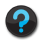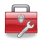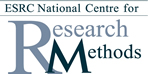# Using Statistical Regression Methods in Education Research# Modules

If you would like to print out our modules you can access the PDFs here.

Module 1 - A Foundation in Statistics

This module provides a basic introduction to statistics and to using SPSS. Topics include sampling, probability, the normal distribution, T-tests, creating variables on SPSS, and making tables and graphs. If you have some experience in these areas you may want to skip this module or perhaps just dip in to it for reference or revision.

Module 2 - Simple Linear Regression

This module introduces the basic concepts underlying regression. Simple linear regression models are a good place to start as they are based on an association between only two variables. This module discusses correlation and crosstabs as well as how a linear model is constructed. It shows you how to run a simple linear regression using SPSS and how to interpret the output.

Module 3 - Multiple Linear Regression

This module expands on our burgeoning knowledge of linear regression by discussing models which include multiple explanatory variables. Interaction effects, the use of different levels of data and 'dummy variables' are discussed in depth. Once again, the module focuses on the practicalities of performing multiple linear regression analysis using SPSS and interpreting the output.

Module 4 - Binary Logistic Regression

This module discusses a type of regression for use with outcomes that can take only two possible values (binary outcomes). Several new concepts required for the understanding of logistic regression are explained, including log functions, odds ratios and log-likelihoods. The procedure for completing a logistic regression analysis using SPSS is explained.

Module 5 - Ordinal Regression

This module builds upon the previous one by describing the process for creating regression models with ordinal outcome variables (outcome variables which are divided in to categories that have a logical order). As before this is explained firmly within the pragmatic context of using SPSS for education research.

 HomeSite GuideResources